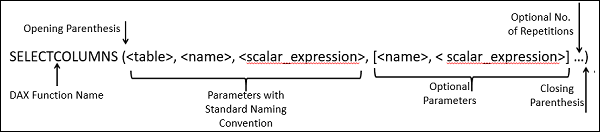DAX Functions - Description Structure

If you have to use a DAX function in a DAX formula, you need to understand the function in detail. You should know the syntax of the function, the parameter types, what the function returns, etc.

To enable you to understand how to read and interpret the DAX functions, a uniform function description structure is used in this tutorial.

• The different types of DAX functions are grouped by the type name of the DAX functions as chapters.

• Each of these chapters provides a brief description of the utility of the respective type of DAX functions.

• The brief description will be followed by the list of DAX functions corresponding to that chapter (Type/Category of DAX functions).

• Each DAX function name is hyperlinked to DAX function details that have the following DAX function description structure −

• Description
• Syntax
• Parameters
• Return Value
• Remarks
• Example

The following sections explain each of these headings that appear in each DAX function explanation.

Description

In the Description section, you will learn what the DAX function is about and where it can be used.

If the DAX function is introduced in Excel 2016, the same will be mentioned here. (Rest of the DAX functions exist in Excel 2013.)

Syntax

In the Syntax section, you will learn the exact function name and the respective parameters.

• DAX function name is given in UPPERCASE letters.

• DAX function name is followed by opening parenthesis.

• Each parameter follows standard DAX parameter naming convention and is enclosed in angle brackets.

• If a parameter is optional, it is further enclosed in square brackets.

• The parameters are separated by commas.

• Ellipses … are used to show an optional number of repetitions of parameters.

• The function syntax ends with closing parenthesis.

ExampleParameters

In the Parameters section, each of the parameters of the specific DAX function is listed in a table with its description. For example, the parameters of the above example DAX function SELECTCOLUMNS is listed in the following table.

Sr.No. Parameter & Description
1

Table

Table or a DAX expression that returns a table.

2

Name

The name given to the column, enclosed in double quotes.

3

scalar_expression

DAX expression that returns a scalar value like a column reference, integer, or string value.

Return Value

In the Return Value section, you will learn about what value the DAX function will return and its data type.

Remarks

In the Remarks section, you will learn about any extra information that you need to know about the usage of the DAX function. You will also understand the potential errors and the reasons.

Example

An example of the usage of the DAX function is given in this section.

Note − When you write DAX functions with the data values for the parameters, you will follow the naming conventions as given below −

• A Table name is specified as it appears in the Data Model. E.g. Sales.

• A Column name is specified as it appears in the Data Model with square brackets enclosing it.

For example, [Sales Amount]

• It is recommended to use fully qualified names for columns, i.e. a column name is prefixed with the table name that contains it.

For example, Sales[Sales Amount]

• If the table name contains spaces, it should be enclosed in single quotes.

For example, ‘East Sales’[Sales Amount]

• A DAX function can return a column or table of values, in which case, it needs to be used as a parameter of another DAX function that requires a column or table.

Useful Video Courses

Video

Mastering DAX and Data Models in Power BI Desktop

53 Lectures 5.5 hours

Video

Mastering DAX Studio

Featured

24 Lectures 2 hours

Video

DAX / Power BI - Customer and Sales Analysis Deep Dive

26 Lectures 4.5 hours